# 「机器学习-李宏毅」:Semi-supervised Learning

## Introduction

Supervised learning（监督式学习）

Semi-supervised learning（半监督式学习）

• Transductive learing: unlabeled data is the testing data. 即这里用来训练的 $\{x^u\}_{u=R}^{R+U}$ 就是来自测试数据集中的数据。（只使用他的feature，而不使用他的label！）
• Inductive learning: unlabeled data is not the testing data.即用来训练的 $\{x^u\}_{u=R}^{R+U}$ 不是来自测试数据集中的数据，是另外的unlabeled data。
• 这里的使用testing data是指使用testing data的feature，即unlabel而不是使用testing data的label。

• Collecting data is easy, but collecting “labelled” data is expensive.

【收集数据很简单，但收集有label的数据很难】

• We do semi-supervised learning in our lives

【在生活中，更多的也是半监督式学习，我们能明白少量看到的事物，但看到了更多我们不懂的，即unlabeled data】

## Why Semi-supervised learning helps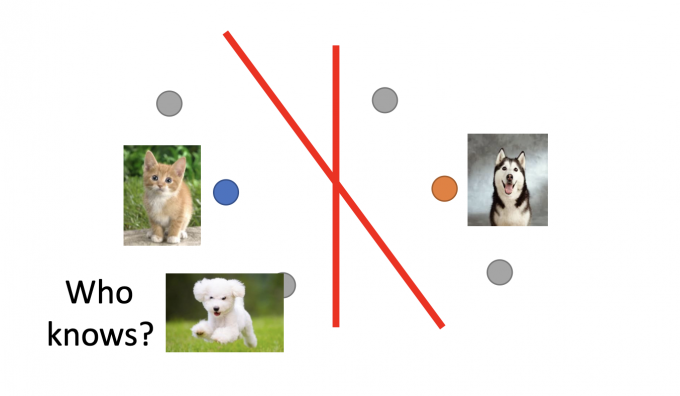The distribution of the unlabeled data tell us something.

# Semi-supervised Learning for Generative Model

## Supervised Generative Model

prior probability（先验概率）有 $P(C_i)$ 和 $P(x|C_i)$ ，假设是Gaussian模型，则 $P(x|C_i)$ 由Gaussian模型中的 $\mu^i,\Sigma$ 参数决定。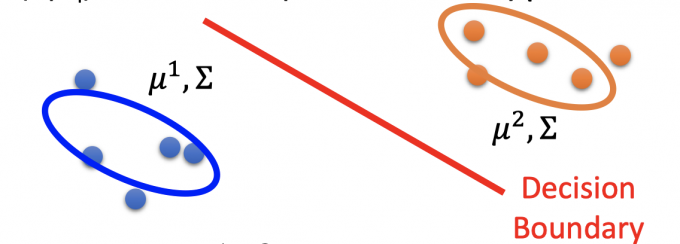## Semi-supervised Generative Model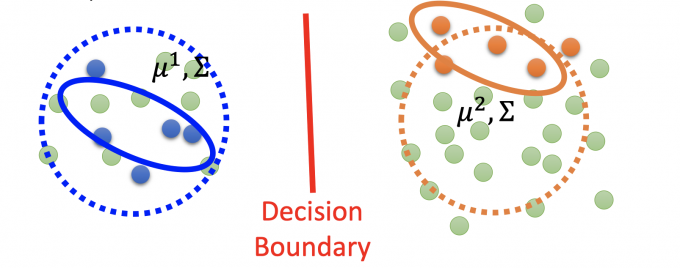The unlabeled data $x^u$ help re-estimate $P(C_1),P(C_2),\mu^1,\mu^2,\Sigma$ .

### EM

1. Initialization : $\theta={P(C_1),P(C_2),\mu^1,\mu^2,\Sigma}$ .

初始化Gaussian模型参数，可以随机初始，也可以通过labeled data得出。

2. E：Step 1: compute the posterior probability of unlabeled data $P_\theta(C_1|x^u)$ (depending on model $\theta$ )

根据当前model的参数，计算出unlabeled data的posterior probability $P(C_1|x^u)$ .(以$P(C_1|x^u)$ 为例)

3. M：Step 2: update model. Back to step1 until the algorithm converges enventually.

用E步得到unlabeled data的posterior probability来最大化极大似然函数，更新得到新的模型参数，公式很直觉。(以 $C_1$ 为例)

（$N$ ：data 的总数，包括unlabeled data; $N_1$ :label= $C_1$ 的data数）

• $P(C_1)=\frac{N_1+\Sigma_{x^u}P(C_1|x^u)}{N}$

对比没有unlabeled data之前的式子， $P(C_1)=\frac{N_1}{N}$ ，除了已有label= $C_1$ ，还多了一部分，即unlabeled data中属于 $C_1$ 的概率和。

• $\mu^{1}=\frac{1}{N_{1}} \sum_{x^{r} \in C_{1}} x^{r}+\frac{1}{\sum_{x^{u}} P\left(C_{1} \mid x^{u}\right)} \sum_{x^{u}} P\left(C_{1} \mid x^{u}\right) x^{u}$

对比没有unlabeled data的式子 ，$\mu^{1}=\frac{1}{N_{1}} \sum_{x^{r} \in C_{1}} x^{r}$ ，除了已有的label= $C_1$ ，还多了一部分，即unlabeled data的 $x^u$ 的加权平均（权重为 $P(C_1\mid x^u)$ ，即属于 $C_1$ 的概率）。

• $\Sigma$ 公式也包括了unlabeled data.

Step 2就是EM算法的Maximum部分，利用unlabeled data（通过已有模型的参数）最大化E步的极大似然函数，更新模型参数。

### Why EM

挖坑EM详解。

• 只有labeled data

极大似然函数 $\log{L(\theta)}=\sum_{x^r}\log{P_\theta(x^r,\hat{y}^r)}$ , 其中 $P_\theta(x^r,\hat{y}^r)=P_\theta(x^r\mid \hat{y}^r)P(\hat{y}^r)$ .

对上式子求导是有closed-form solution的。

• 有labeled data和unlabeled data

极大似然函数增加了一部分 $\log L(\theta)=\sum_{x^{r}} \log P_{\theta}\left(x^{r}, \hat{y}^{r}\right)+\sum_{x^{u}} \log P_{\theta}\left(x^{u}\right)$ .

将后部分用全概率展开， $P_{\theta}\left(x^{u}\right)=P_{\theta}\left(x^{u} \mid C_{1}\right) P\left(C_{1}\right)+P_{\theta}\left(x^{u} \mid C_{2}\right) P\left(C_{2}\right)$ .

如果要求后部分，因为是unlabeled data, 所以模型 $\theta$ 需要得知unlabeled data的label，即 $P(C_1\mid x^u)$ ,而求这个式子，也需要得到 prior probability $P(x^u\mid C_1)$ ,但这个式子需要事先得知模型 $\theta$ ，因此陷入了死循环。

因此这个极大似然函数不是convex（凸），不能直接求解，因此用迭代的EM算法逐步maximum极大似然函数。

# Low-density Separation Assumption

## Self-training

Given：labeled data set $=\{(x^r,\hat{y}^r\}_{r=1}^R$ ,unlabeled data set $=\{x^u\}_{u=R}^{R+U}$ .

Repeat：

1. Train model $f^*$ from labeled data set. $f^*$ is independent to the model)

从labeled data set中训练出一个模型

2. Apply $f^*$ to the unlabeled data set. Obtain pseudo-label $\{(x^u,y^u\}_{u=l}^{R+U}\}$

用这个模型 $f^*$ 来预测unlabeled data set， 获得伪label

3. Remove a set of data from unlabeled data set, and add them into the labeled data set.

拿出一些unlabeled data(pseudo-label)，放到labeled data set中，回到步骤1，再训练。

• how to choose the data set remains open

如何选择unlabeled data 是自设计的

• you can also provide a weight to each data.

训练中可以对unlabeled data(pseudo-label)和labeled data 赋予不同的权重.

## Hard Label

V.S. semi-supervised learning for generative model

Semi-supervised learning for generative model和Low-density Separation的区别其实是soft label 和hard label的区别。

Generative Model是利用来unlabeled data的 $P(C_1|x^u)$ posterior probability来计算新的prior probability，迭代更新模型。

($\theta^*$ 是labeled data计算所得的network parameters)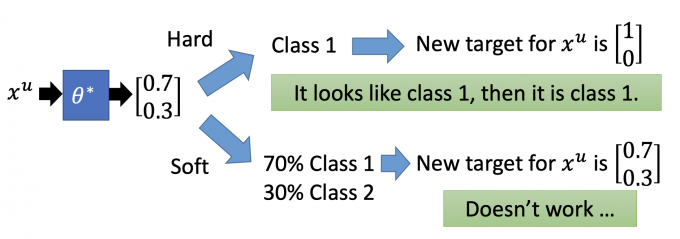## Entropy-based Regularization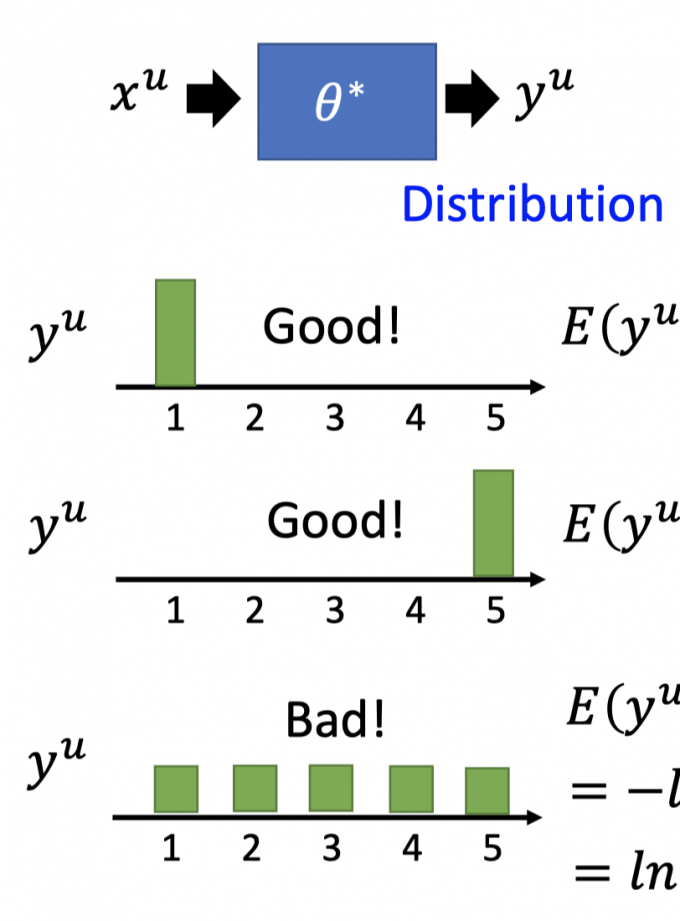(注：这里的 $y^u_m$ 是变量 $y^u=m$ 的概率)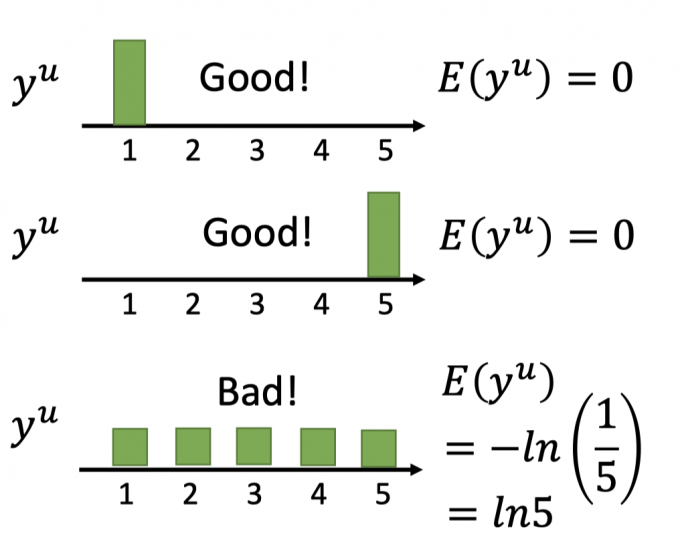$L=\sum_{y^r} C(x^r,\hat{y}^r)+\lambda\sum_{x^u}E(y^u)$

Loss function的前一项（cross entropy）minimize保证分类的正确性，后一项（entropy of $y^u$ ) minimize保证 unlabeled data分布尽量集中，最大可能满足low-density separation的假设。

## Outlook: Semi-supervised SVM

SVM也是解决semi-supervised learning的方法.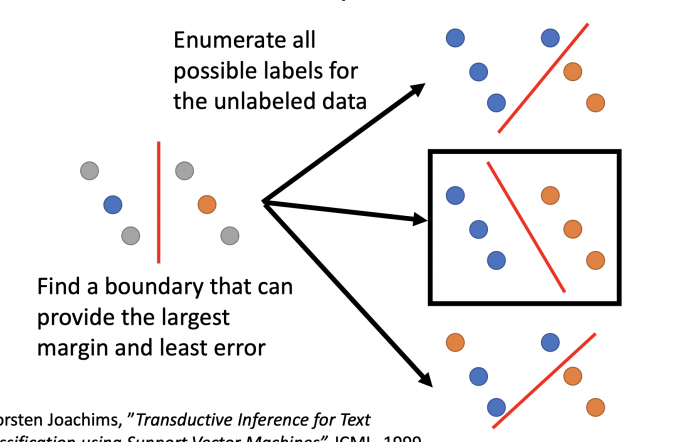# Smoothness Assumption

Smoothness Assumption的思想可以用以下话归纳：

“You are known by the company you keep”

Assumption：“similar” $x$ has the same $\hat{y}$ .

【意思就是说：相近的 $x$ 有相同的label $\hat{y}$ .】

More precise assumption：

• x is not uniform
• if $x^1$ and $x^2$ are close in a hign density region, $\hat{y}^1$ and $\hat{y}^2$ are the same.

Smoothness Assumption假设更准确的表述是：

x不是均匀分布，如果 $x^1$ 和 $x^2$ 通过一个high density region的区域连在一起，且离得很近，则 $\hat{y}^1$ 和 $\hat{y}^2$ 相同。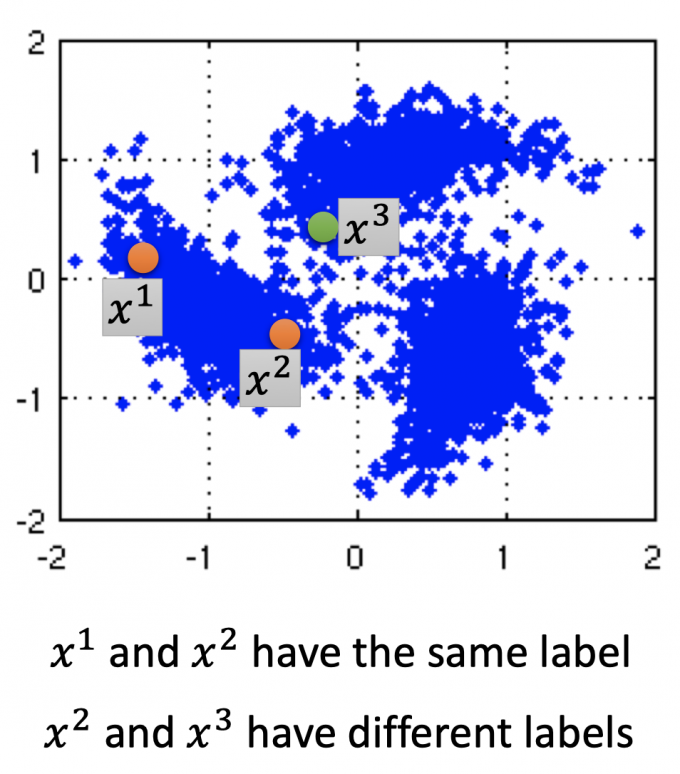Smoothness Assumption通过观察大量unlabeled data，可以得到一些信息。

Smoothness Assumption还可以用在文章分类中，比如分类天文学和旅游学的文章。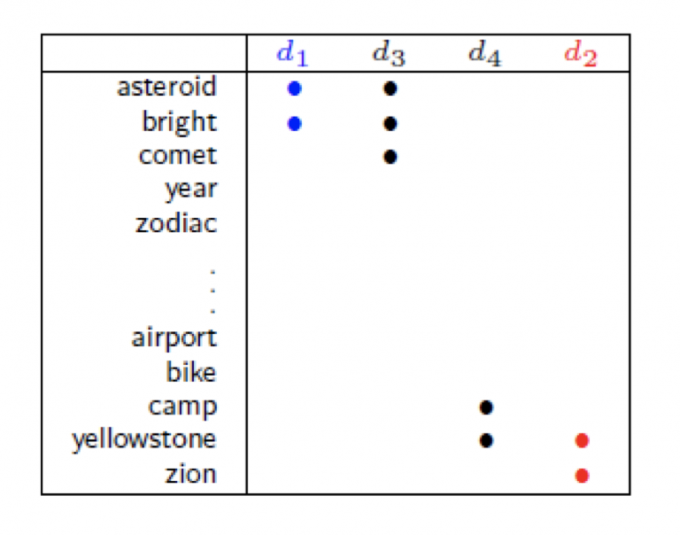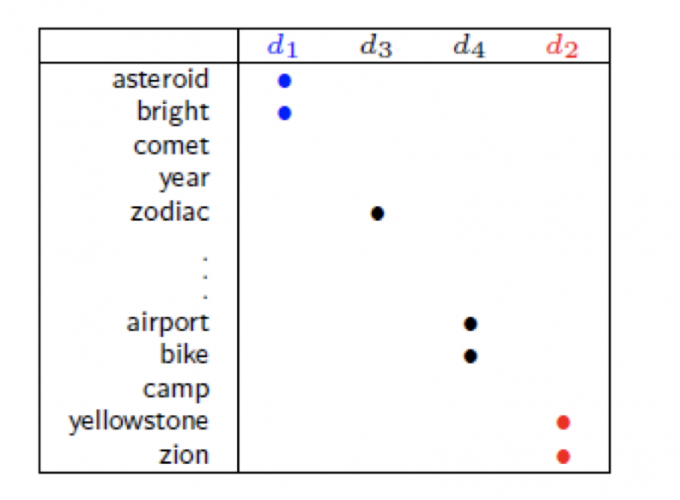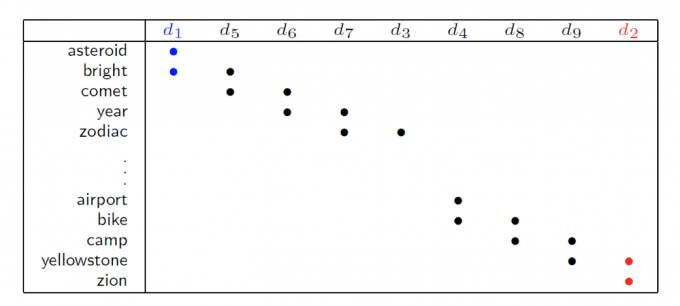## Graph-based Approach

Represent the data points as a graph.

【把这些数据点看作一个图】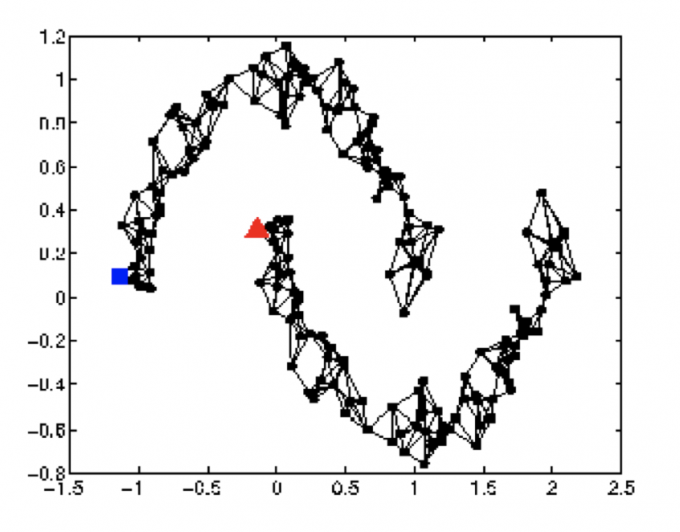### Graph Construction

1. Define the similarity $s(x^i, x^j)$ between $x^i$ and $x^j$ .

【定义data $x^i$ 和 $x^j$ 的相似度】

• K Nearest Neighbor【和该点最近的k个点相连接】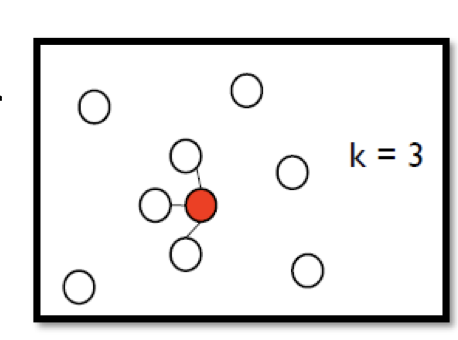• e-Neighborhood【与离该点距离小于等于e的点相连接】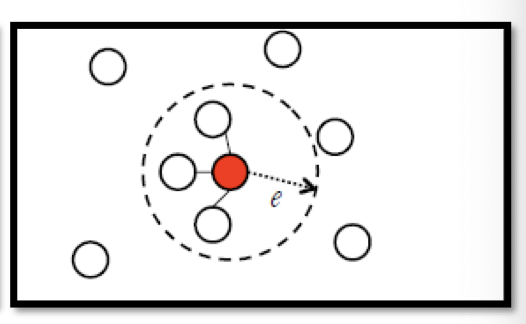3. Edge weight is proportional to $s(x^i, x^j)$ 【边点权重就是步骤1定义的连接两点的相似度】

Gaussian Radial Basis Function： $s\left(x^{i}, x^{j}\right)=\exp \left(-\gamma\left\|x^{i}-x^{j}\right\|^{2}\right)$

一般采用如上公式（经验上取得较好的performance）。

因为利用指数化后（指数内是两点的Euclidean distance），函数下降的很快，只有当两点离的很近时，该相似度 $s(x^i,x^j)$ 才大，其他时候都趋近于0.

### Graph-based Approach

The labeled data influence their neighbors.

Propagate through the graph.

【label data 不仅会影响他们的邻居，还会一直传播下去】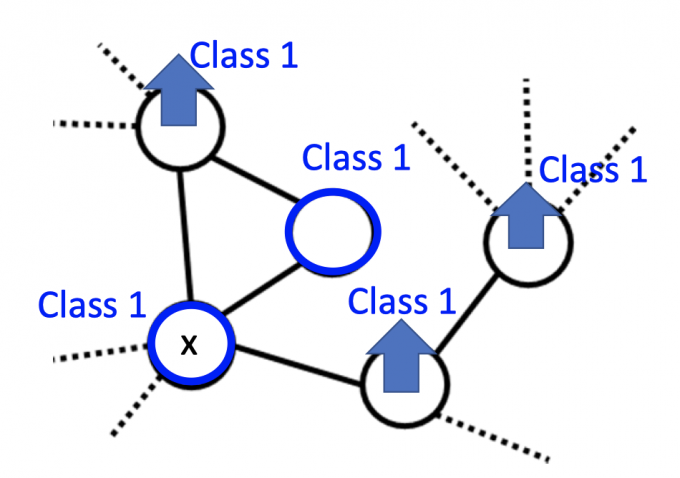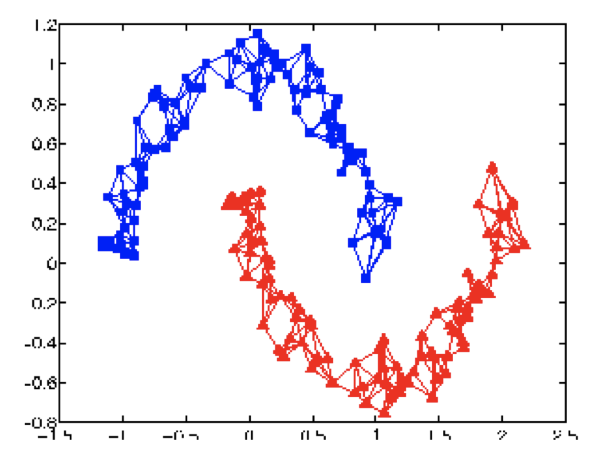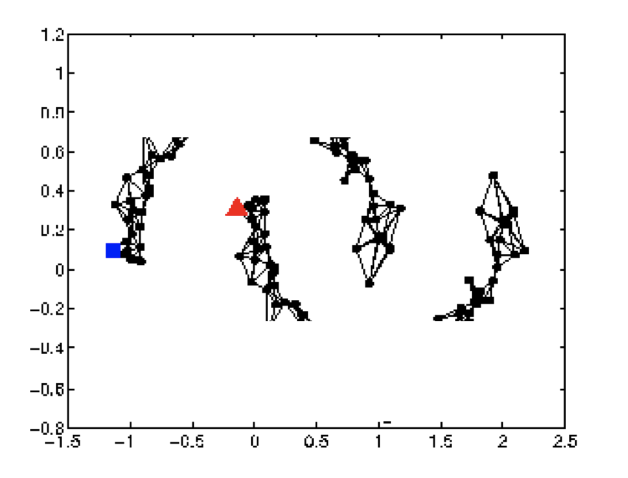### Smoothness Definition

$S=\frac{1}{2} \sum_{i,j} w_{i, j}\left(y^{i}-y^{j}\right)^{2}$

（对于所有的labeled data 和 “unlabeled data”（作为NN输入后，有label））

Smaller means smoother.

【Smoothness $S$ 越小，表示图越满足这个假设】

$S=\frac{1}{2} \sum_{i, j} w_{i, j}\left(y^{i}-y^{j}\right)^{2}=y^{T} L y$ (这里的1/2只是为了计算方便)
• $y$ : (R+U)-dim vector，是所有label data和”unlabeled data” 的label，所以是R+U维。

$y=\begin{bmatrix}…y^i…y^j…\end{bmatrix}^T$

• $L$ :(R+U) $\times$ (R+U) matrix，也叫Graph Laplacian（调和矩阵，拉普拉斯矩阵）

$L$ 的计算方法：$L=D-W$

其中 $W$ 矩阵算是图的邻接矩阵（区别是无直接可达边的值是0）

$D$ 矩阵是一个对角矩阵，对角元素的值等于 $W$ 矩阵对应行的元素和

矩阵表示如下图所示：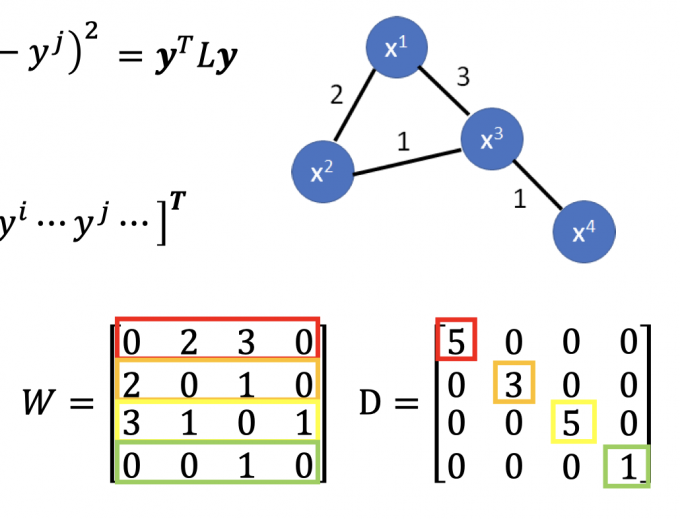（证明据说很枯燥，暂时略)

### Smoothness Regularization

$S=\frac{1}{2} \sum_{i, j} w_{i, j}\left(y^{i}-y^{j}\right)^{2}=y^{T} L y$

$S$ 中的 $y$ 其实是和network parameters有关的（unlabeled data的label），所以把 $S$ 也放进损失函数中minimize，以求尽可能满足smoothness assumption.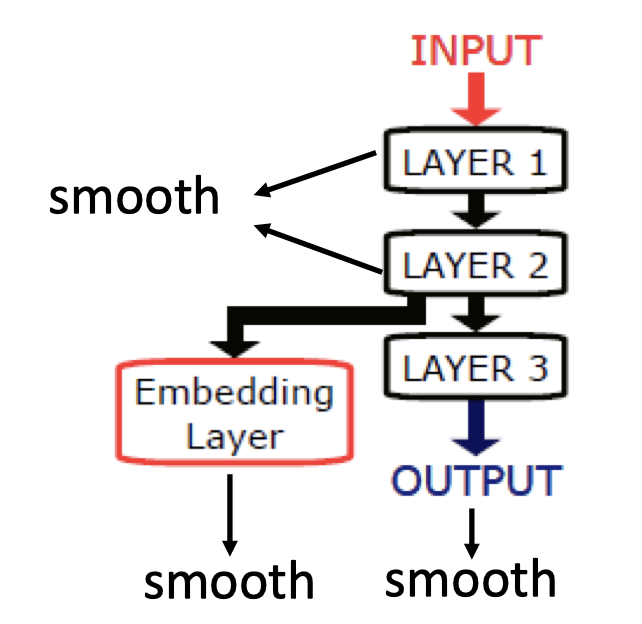# Better Representation

Better Presentation的思想就是：去芜存菁，化繁为简。

• Find the latent(潜在的) factors behind the observation.
• The latent factors (usually simpler) are better representation.

【找到所观察事物的潜在特征，即该事物的better representation】

# Reference

1. 挖坑：EM算法详解

2. 挖坑：Graph Laplacian in smoothness.

3. Olivier Chapelle：Semi-Supervised Learning

「机器学习-李宏毅」:Semi-supervised Learning

https://f7ed.com/2020/07/03/semi-supervised/

f1ed

2020-07-03

2021-01-30